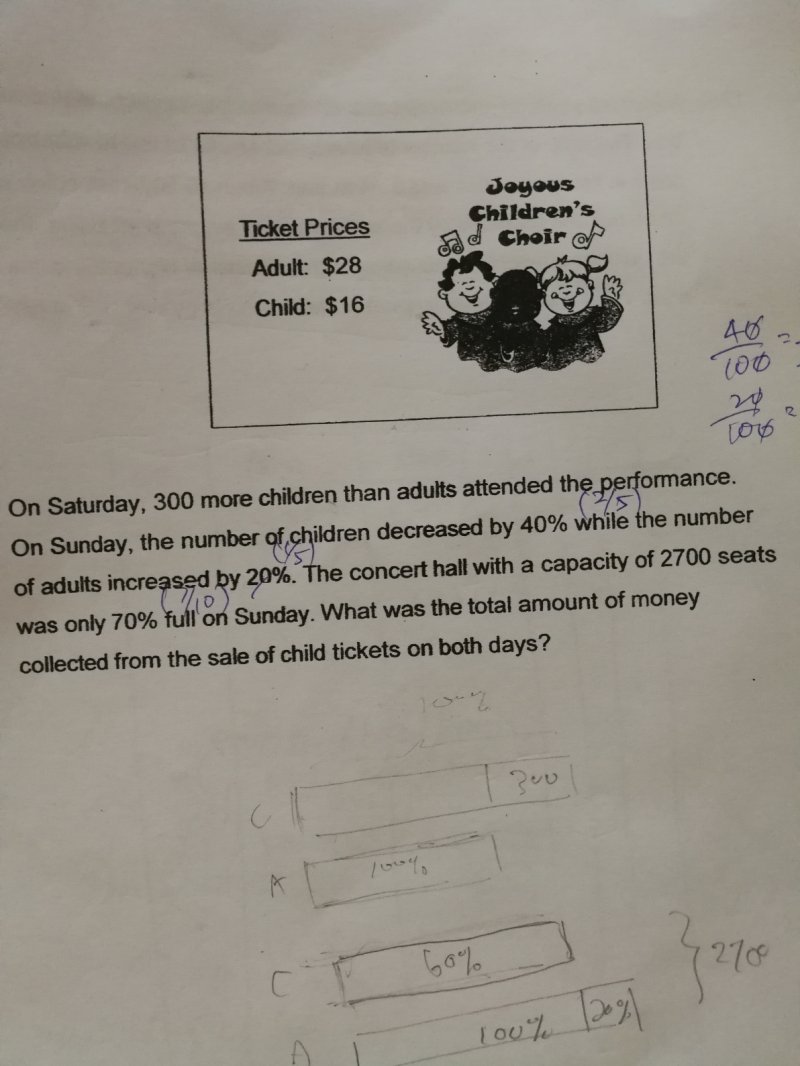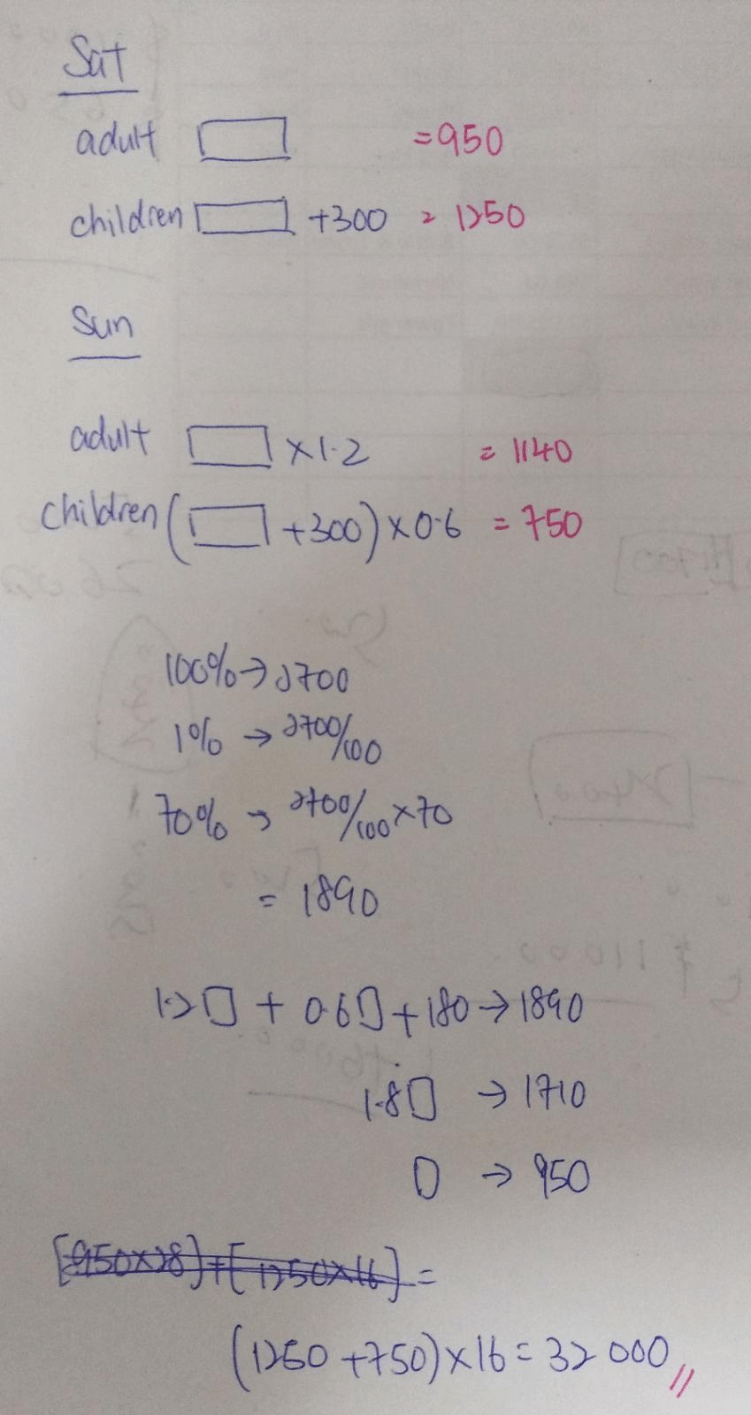# QuestionHi,

Need help on this question !

Appreciate someone who can help.

Thanks.0 Replies 0 Likes

Saturday —
adults : 100 units
children : 100 units + 300

Sunday —
adults : 100 units x (100% + 20%) = 120 units
children : 100 units + 300 x (100% – 40%) = 60 units + 180

2700 x 70% = 1890 (seats filled on Sunday)
120 units + 60 units + 180 = 180 units + 180 = 1890
1 unit = (1890 – 180)/180 = 9.5
100 units + 300 + 60 units + 180 = 160 units + 480 = 160 x 9.5 + 480 = 2000
2000 x 16 = 32000

Ans : \$32000.

0 Replies 0 Likes